# SAT Math Multiple Choice Question 828: Answer and Explanation

### Test Information

Question: 828

3. An architect is working on a design for a solar power plant. While double-checkingthe blueprint, he realizes that he has made an error: The solar panels will not fiton the roof unless the dimensions of the roof are increased by 0.5%. Which of thefollowing expresses this change in the dimensions of the roof as a fraction of theoriginal dimensions?

• A.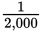• B.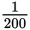• C.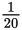• D.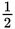Getting to the Answer: Reword the question in your head: 0.5% is what fraction of the original dimensions? In other words, this question is simply asking you to convert 0.5% to a fraction. (Don't answer too quickly, though:is 0.5, not 0.5%, so D is not correct.) Recall that "percent" means per hundred, which translates to divide by 100. Therefore,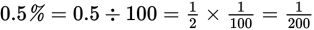. The answer is (B).